Question

# A box slides down an inclined plane with an acceleration that is precisely two-fifths what it...

A box slides down an inclined plane with an acceleration that is precisely two-fifths what it would have been if the slide had been frictionless. Calculate the angle of the incline if the coefficient of kinetic friction of the rough incline is 0.29.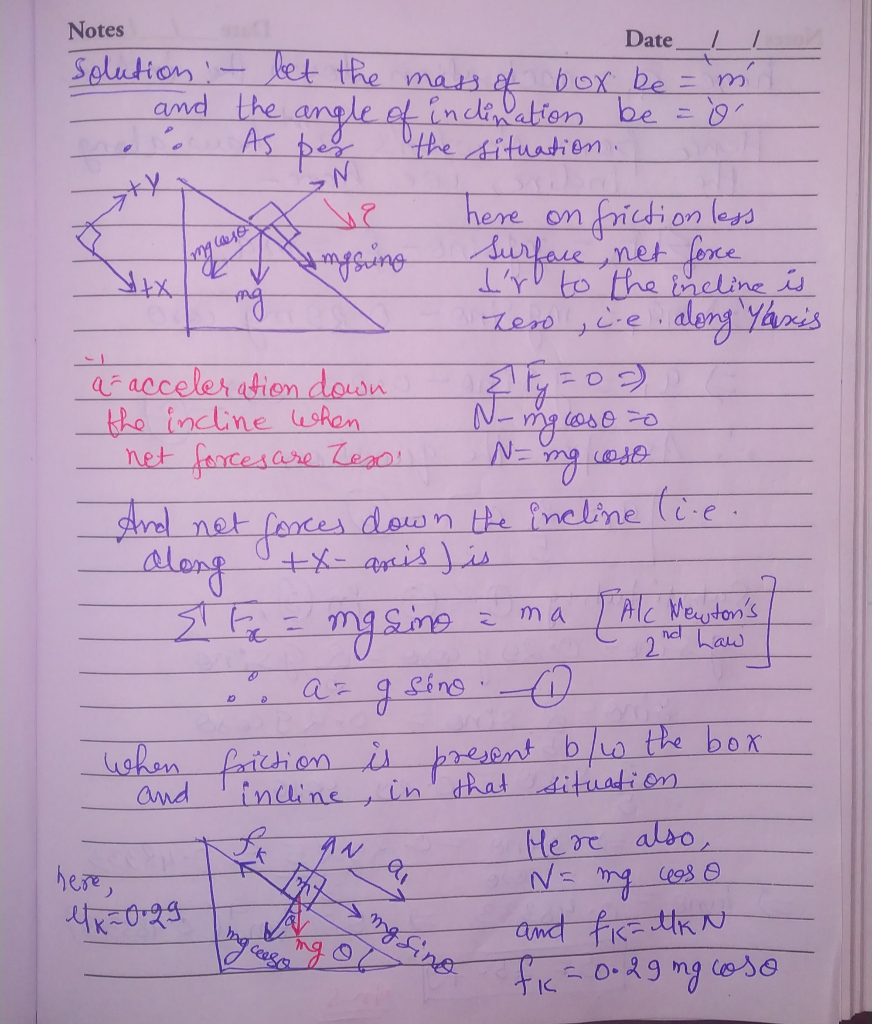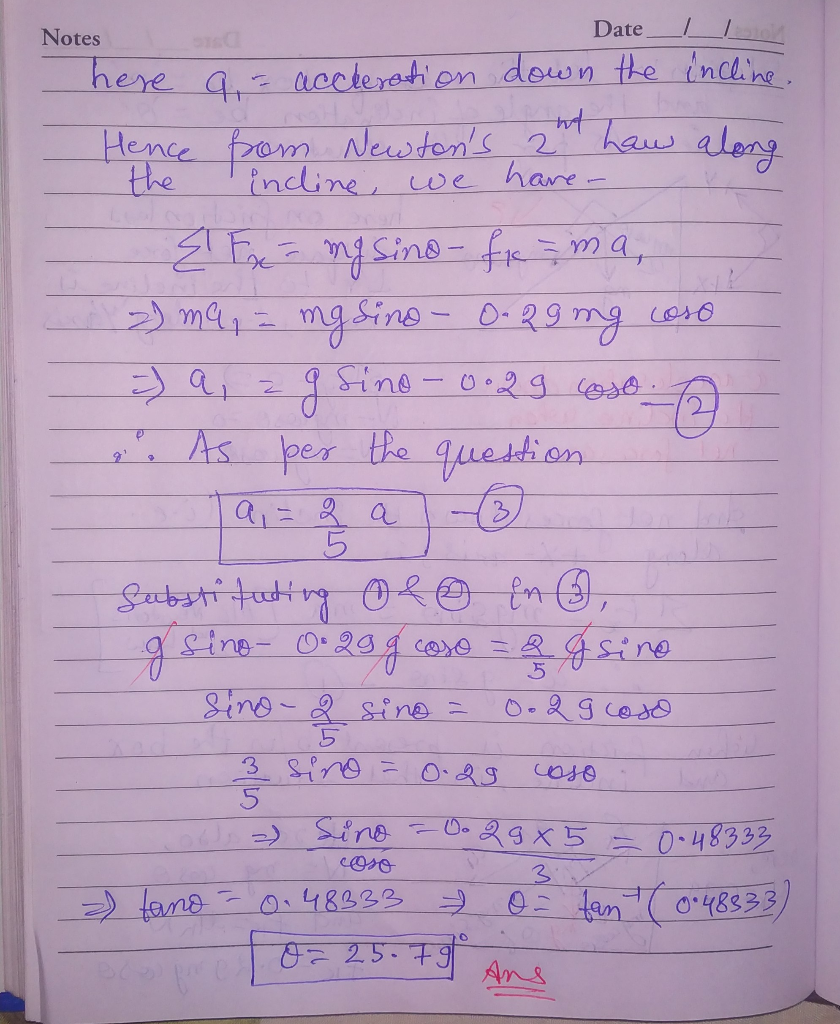#### Earn Coins

Coins can be redeemed for fabulous gifts.

Similar Homework Help Questions
• ### A child slides down a slide with a 20 degree incline, and at the bottom her...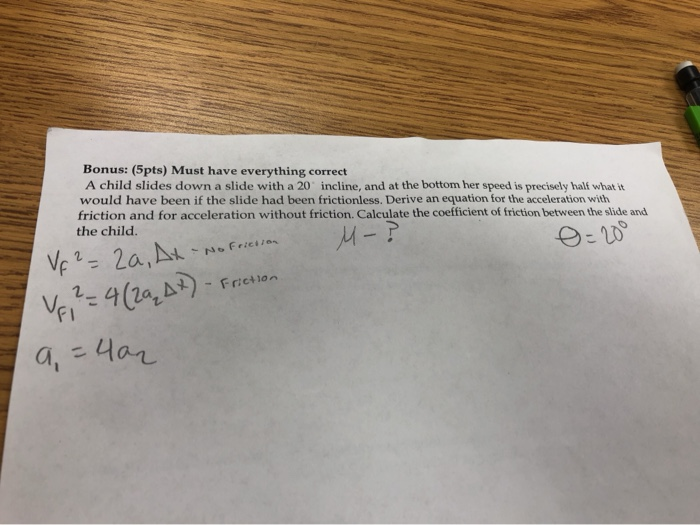A child slides down a slide with a 20 degree incline, and at the bottom her speed is percisely half what it would have been if the slide was frictionless. Derive an equation for the acceleration with friction and for acceleration without friction. Calculate the coefficient of friction between the slide and the child. Bonus: (5pts) Must have everything correct A child slides down a slide with a 20 incline, and at the bottom her speed is precisely half what...

• ### A 1.0 kg box starts from rest and slides down a very rough inclined plane as...

A 1.0 kg box starts from rest and slides down a very rough inclined plane as shown below. Use energy principles to determine the coefficient of friction on the incline surface if its speed at the bottom is 2.78 m/s. The box sits on a incline that is 0.5m above the ground on a 30 degree incline.

• ### Part A A 6.0 kg box slides down an inclined plane that makes an angle of...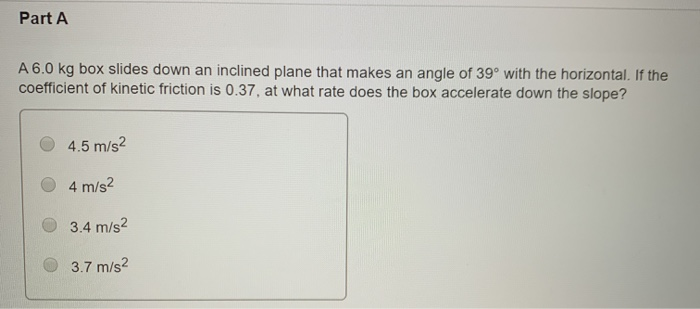Part A A 6.0 kg box slides down an inclined plane that makes an angle of 39° with the horizontal. If the coefficient of kinetic friction is 0.37, at what rate does the box accelerate down the slope? 4.5 m/s2 4 m/s2 3.4 m/s2 3.7 m/s2

• ### As shown in the figure, a block weighing 150 NN slides down a plane inclined at...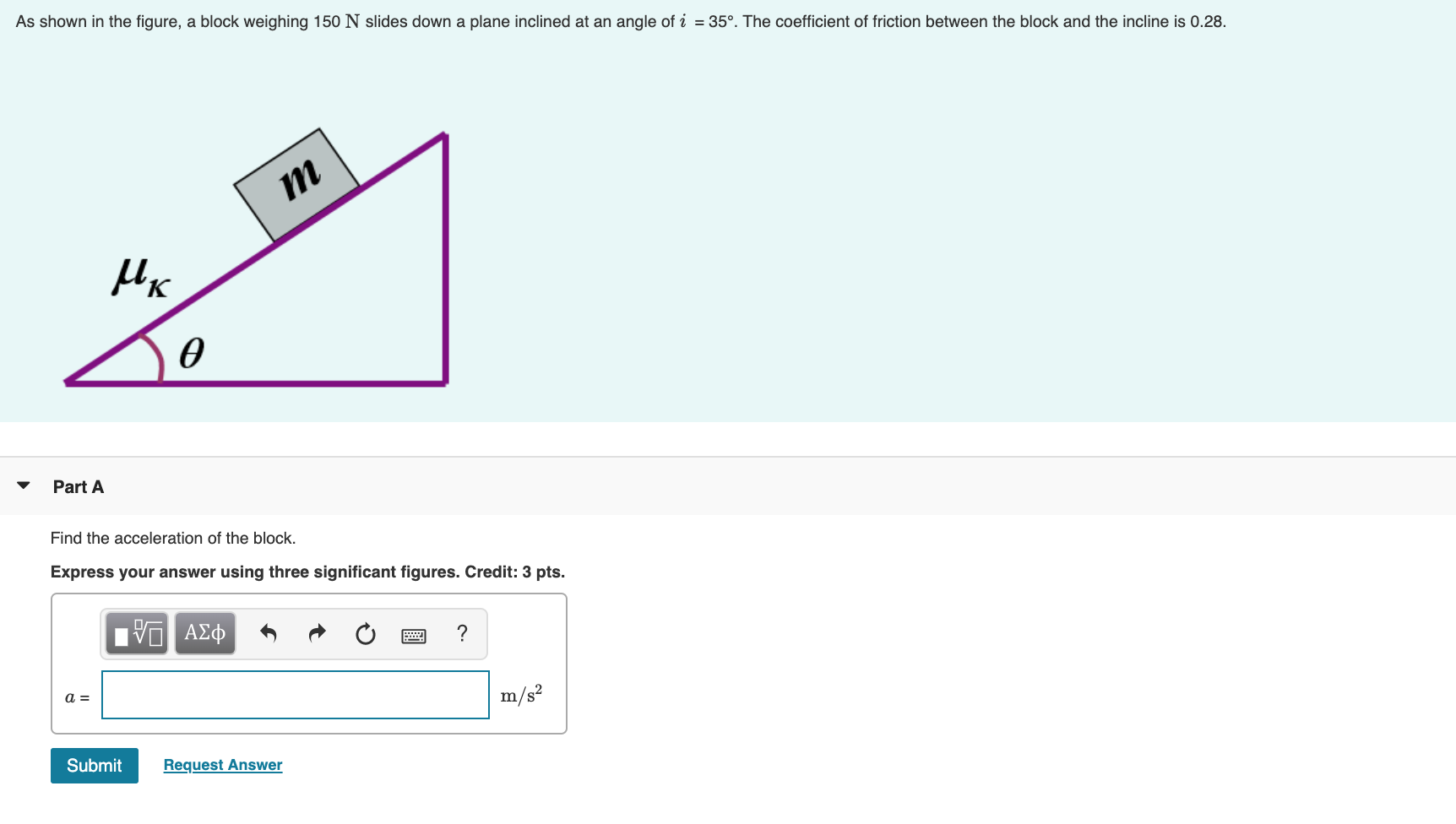As shown in the figure, a block weighing 150 NN slides down a plane inclined at an angle of iθ = 35﻿°. The coefficient of friction between the block and the incline is 0.28. As shown in the figure, a block weighing 150 N slides down a plane inclined at an angle of i = 35º. The coefficient of friction between the block and the incline is 0.28. m их e Part A Find the acceleration of the block. Express...

• ### A box of weight w=2.0N accelerates down a rough plane that is inclined at an angle...

A box of weight w=2.0N accelerates down a rough plane that is inclined at an angle ϕ=30∘ above the horizontal, as shown (Figure 6) . The normal force acting on the box has a magnitude n=1.7N, the coefficient of kinetic friction between the box and the plane is μk=0.30, and the displacement d⃗ of the box is 1.8 m down the inclined plane. What is the work Wfk done on the box by the force of kinetic friction?​

• ### A skier of mass m started at rest and slides down over a frictionless inclined plane....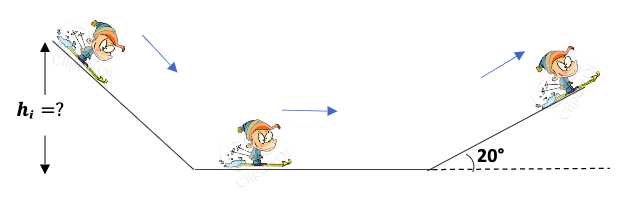A skier of mass m started at rest and slides down over a frictionless inclined plane. He reached the bottom at a speed of 20 m/s. Then reached the second inclined plan. If the second inclined plane has a friction coefficient of 0.2 and inclined at angle of 20°. How far does he slide on it before coming momentarily to rest? hi =? 20°

• ### Mi A mass M slides-downward along a rough plane surface inclined at angle = 29.21 in...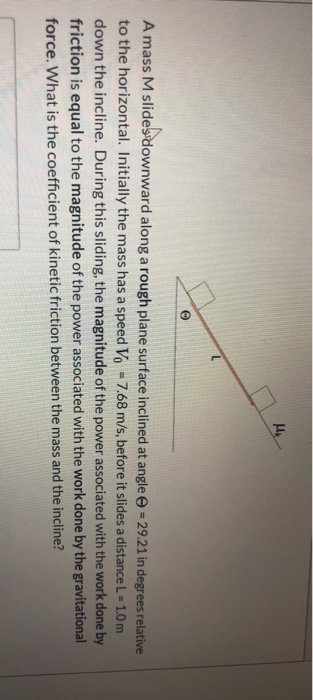Mi A mass M slides-downward along a rough plane surface inclined at angle = 29.21 in degrees relative to the horizontal. Initially the mass has a speed Vo = 7.68 m/s, before it slides a distance L = 1.0 m down the incline. During this sliding, the magnitude of the power associated with the work done by friction is equal to the magnitude of the power associated with the work done by the gravitational force. What is the coefficient of...

• ### A box, mass m, slides without friction down an incline plane at an angle θ with...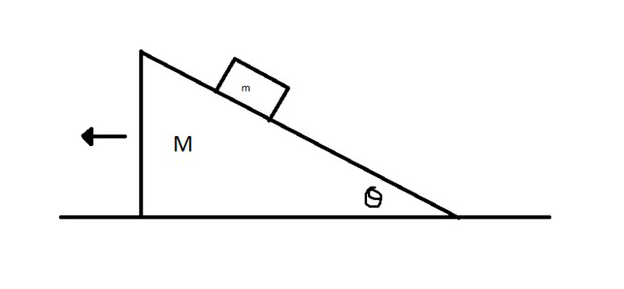A box, mass m, slides without friction down an incline plane at an angle θ with the horizontal. The incline plane is free to slide without friction across the floor it is resting on. Find the vector acceleration of both the plane and the box with respect to the floor. Confirm your answer using both Conservation of Energy and Newton’s Laws.

• ### A mass M slides downward along a rough plane surface inclined at angle \Theta\: Θ =...

A mass M slides downward along a rough plane surface inclined at angle \Theta\: Θ = 29.8 in degrees relative to the horizontal. Initially the mass has a speed V_0\: V 0 = 5.32 m/s, before it slides a distance L = 1.0 m down the incline. During this sliding, the magnitude of the power associated with the work done by friction is equal to the magnitude of the power associated with the work done by the gravitational force. What...

• ### A mass M slides downward along a rough plane surface inclined at angle \Theta\: Θ =...

A mass M slides downward along a rough plane surface inclined at angle \Theta\: Θ = 31.7 in degrees relative to the horizontal. Initially the mass has a speed V_0\: V 0 = 6.9 m/s, before it slides a distance L = 1.0 m down the incline. During this sliding, the magnitude of the power associated with the work done by friction is equal to the magnitude of the power associated with the work done by the gravitational force. What...